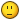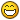## Recommended Posts

So i have 10 sprites. They should follow each other in a line around the map. Instead they all turn when the first one hits the bounds check. You can see what i am talking about here:

http://www.kobaltic.com/dcor/

They should all be in one line and follow the path. It works correctly with one sprite but not all of them. here is the code.

This is called in the update to move the sprites

` for(var i=0;i<10;i++){                moveEnemy(gTroll[i]);            }`

Theses are the create and move functions

`function createEnemies() {    var trollEastArray = [0, 1, 2, 3, 4, 5, 6, 7];    var trollNorthArray = [8, 9, 10, 11, 12, 13, 14, 15];    var trollSouthArray = [32, 33, 34, 35, 36, 37, 38, 39];    var trollWestArray = [57, 58, 59, 60, 61, 62, 63, 64];        for (var i=0;i<10;i++){       xCor = 100 * -1 *(i +1) ;    gTroll[i] = game.add.sprite(xCor,30, 'gTroll'); //50,30    gTroll[i].animations.add('gTrollEast', trollEastArray);    gTroll[i].animations.add('gTrollWest', trollWestArray);    gTroll[i].animations.add('gTrollNorth', trollNorthArray);    gTroll[i].animations.add('gTrollSouth', trollSouthArray);             game.physics.enable(gTroll[i]);    gTroll[i].debug = true;       gTroll[i].checkWorldBounds = true;      }}function moveEnemy(sprite){    var speed = 1;   // debugMessage.innerHTML = count1;     sprite.x = sprite.x + xValue * speed;    sprite.y = sprite.y + yValue * speed;    sprite.animations.play(dir,10,true);    followPath(sprite);  }function followPath(sprite){   var bounds = sprite.getBounds();   if (Phaser.Rectangle.intersects(bounds, point1)){       xValue = 0;       yValue = 1;       dir = 'gTrollSouth';       test = 1;   }  if (Phaser.Rectangle.intersects(bounds, point2)){       xValue = -1;       yValue = 0;        dir = 'gTrollWest';        test = 2;   }  if  (Phaser.Rectangle.intersects(bounds, point3)){       xValue = 0;       yValue = 1;        dir = 'gTrollSouth';        test = 3;   }   if  (Phaser.Rectangle.intersects(bounds, point4)){       xValue = 1;       yValue = 0;        dir = 'gTrollEast';        test =4;   }  if  (Phaser.Rectangle.intersects(bounds, point5)){       xValue = 0;       yValue = 1;        dir = 'gTrollSouth';        test = 5;   }   if  (Phaser.Rectangle.intersects(bounds, point6)){       xValue = 1;       yValue = 0;        dir = 'gTrollEast';        test = 6;   }   if  (Phaser.Rectangle.intersects(bounds, point7)){       xValue = 0;       yValue = -1;        dir = 'gTrollNorth';        test = 7;   }  if  (Phaser.Rectangle.intersects(bounds, point8)){       xValue = -1;       yValue = 0;        dir = 'gTrollWest';        test = 8;   }  if  (Phaser.Rectangle.intersects(bounds, point9)){       xValue = 0;       yValue = 1;        dir = 'gTrollSouth';        test =9;   }   }`

Hopefully this is enough code. Thanks for any help.

##### Share on other sites

I can't seem to see the problem specifically (I'm pretty sure I know what the problem is, but not what's causing it) but I think the way you're doing things is inviting problems such as this. A better way to do this would be to extend Phaser.Sprite with your own object which performs the followPath function on its own update loop, something like this (untested but it should work):

`var Troll = function(game, x, y) {  Phaser.Sprite.call(this, game, x, y, 'gTroll');    var trollEastArray = [0, 1, 2, 3, 4, 5, 6, 7];  var trollNorthArray = [8, 9, 10, 11, 12, 13, 14, 15];  var trollSouthArray = [32, 33, 34, 35, 36, 37, 38, 39];  var trollWestArray = [57, 58, 59, 60, 61, 62, 63, 64];    this.animations.add('gTrollEast', trollEastArray);  this.animations.add('gTrollWest', trollWestArray);  this.animations.add('gTrollNorth', trollNorthArray);  this.animations.add('gTrollSouth', trollSouthArray);    game.physics.enable(this);    this.debug = true;  this.checkWorldBounds = true;    // our individual troll properties  this.dir = 'gTrollNorth';  this.xValue = 0;  this.yValue = 0  this.test = 0;  // a local speed so you can change individual trolls in addition to overall speed  this.speed = 1;};Troll.prototype = Object.create(Phaser.Sprite.prototype);Troll.prototype.constructor = Troll;// a 'static' speed setting accessible globally - if you change Troll.speed anywhere else// all trolls will instantly change speed to matchTroll.speed = 1;// this function will be called every frame, just like the update function in your stateTroll.prototype.update = function() {  this.move();  this.followPath();};Troll.prototype.move = function() {  // modified the calculation to take into account individual speed as well  this.x = (this.x + this.xValue * this.speed) * Troll.speed;  this.animations.play(this.dir, 10, true);};Troll.prototype.followPath = function() {  var bounds = this.getBounds();  if (Phaser.Rectangle.intersects(bounds, point1)){    this.xValue = 0;    this.yValue = 1;    this.dir = 'gTrollSouth';    this.test = 1;  }  if (Phaser.Rectangle.intersects(bounds, point2)){    this.xValue = -1;    this.yValue = 0;    this.dir = 'gTrollWest';    this.test = 2;  }  if  (Phaser.Rectangle.intersects(bounds, point3)){    this.xValue = 0;    this.yValue = 1;    this.dir = 'gTrollSouth';    this.test = 3;  }  if  (Phaser.Rectangle.intersects(bounds, point4)){    this.xValue = 1;    this.yValue = 0;    this.dir = 'gTrollEast';    this.test = 4;  }  if  (Phaser.Rectangle.intersects(bounds, point5)){    this.xValue = 0;    this.yValue = 1;    this.dir = 'gTrollSouth';    this.test = 5;  }  if  (Phaser.Rectangle.intersects(bounds, point6)){    this.xValue = 1;    this.yValue = 0;    this.dir = 'gTrollEast';    this.test = 6;  }  if  (Phaser.Rectangle.intersects(bounds, point7)){    this.xValue = 0;    this.yValue = -1;    this.dir = 'gTrollNorth';    this.test = 7;  }  if  (Phaser.Rectangle.intersects(bounds, point8)){    this.xValue = -1;    this.yValue = 0;    this.dir = 'gTrollWest';    this.test = 8;  }  if  (Phaser.Rectangle.intersects(bounds, point9)){    this.xValue = 0;    this.yValue = 1;    this.dir = 'gTrollSouth';    this.test = 9;  }};`

Then to use, just change your createEnemies function to this:

`function createEnemies() {        for (var i=0;i<10;i++) {      xCor = 100 * -1 *(i +1) ;      gTroll[i] = new Troll(game, xCor, 30);      }}`

All of the trolls should then be acting individually. This is a much nicer way to manage things as it means each troll handles its own affairs. I'd recommend looking into object oriented programming in JavaScript; there are plenty of articles about - this is a good one to start with.

##### Share on other sites

For what it's worth, this is pretty verbatim and looking at what you're wanting to do, it may be better also to create maybe special 'direction changer' sprites each with a direction property which are then checked for overlap. Then when a troll overlaps this sprite, the sprite direction is determined and that troll then starts moving in this direction. You'd then just put these sprites at the end of path sections in the appropriate place so the instant the troll touches one, it changes direction.

##### Share on other sites

1. I think my problem is that I have dir, xValue, yValue all Global. (I know that was smart of me)

2. You are right and I need to move to OOP for JS as well. I use it in PHP so why not JS.

3. You are ahead of me with the direction changer. I am going to put in a way point system based off of a way point sprite. Then I can easly manage the locations in TILED.

##### Share on other sites

D'oh, yeah I just saw that now you mention it. This is exactly the sort of thing that OO-style stuff helps avoid##### Share on other sites

I put everything in and got most of it to work. I am getting this error but ran out of time to research it.

TypeError: this.onTextureUpdate is not a function       phaser.....0.5.js (line 3)
##### Share on other sites

After working with this a bit it seems that this call isn't working

`Phaser.Sprite.call(this, game, x, y, 'gTroll');`

I get this error:

`Uncaught TypeError: undefined is not a function`
##### Share on other sites

That's odd, this is just a standard example of extending a sprite, the same as this: http://examples.phaser.io/_site/view_full.html?d=sprites&f=extending+sprite+demo+2.js&t=extending%20sprite%20demo%202

Can you do console.log(Phaser.Sprite) to ensure that it actually exists and is initialised at the point where you're trying to extend it? Though by all accounts it should be.

##### Share on other sites

I had to add in this part to get it to work.

`Troll.prototype = Object.create(Phaser.Sprite.prototype);Troll.prototype.constructor = Troll;`
Oops, my mistake! Sorry, I did say it was untested!